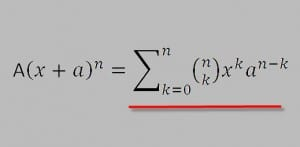# Wrong formulas to Convert Percencetage to GPA 4.0 scale by International students for Applying to US Schools

In Application Info by KumarUpdated : 3 CommentsI have seen many international students getting confused about converting percentage to GPA 4.0 scale. To clarify this question, I have wrote an article before : Should you convert Marks/ Percentage to GPA 4.0 Scale for USA Applications ? But, there are so many students who use some WRONG Methods to convert percentage to GPA.

## Why students use Wrong Formulas to Convert Percentage to GPA 4.0 Scale:

You will not believe how many wrong methods or formulas international students use. In all reality, international students are not aware or confused and are using the formulas that US schools use to compute GPA. It is just a matter of not knowing and generalizing the standards and formulas used in US for doing similar conversion of percentages.  Converting using wrong formulas is as bad as using wrong units. So, do NOT convert.

Let me share with you some of those wrong formulas. If you are international student, please Do NOT use these formulas when applying to US Schools. These are just to WARN you so that you do NOT any of these formulas blindly.

## Wrong Method 1 – Assigning a letter grade :

Directly Assigning a Letter Grade to a percentage. This is a US grading system scale.

 Grade Grade Point Percentage A 4 90 – 100 B 3 80 – 89.9 C 2 70 – 79.9 D 1 60 – 69.9 F 0 <59

## Wrong method 2 – More Finer assignment of letter grade :

A little finer method than above that gives GPA with more precision with decimals of getting grade point and converting percentage to GPA

 Grades Grade Points Percentage of Marks A 4.000 Greater than 93% A- 3.670 87 -89.9% B+ 3.330 84-86.9 % B 3.000 80 – 83.9% B- 2.670 77 – 79.9% C+ 2.330 74 – 76.9% C 2.000 70 – 73.9% C- 1.670 67- 69.9% D+ 1.330 65-66.9% D 1.000 62-64.9% D- 0.670 59-61.9% F 0.000 <59 %

## Wrong  Formula 3 – Concept of Averaging Marks and GPA:

Computing an average of all the subjects Marks and then assign a letter grade for overall GPA.

For instance, say Marks are :  English = 80, C and Data Structures= 90 , Engineering  Mathematics = 70, Physics =70, Engineering Chemistry = 70, IT for Engineers = 80.

Someone would just add these and get average = (80+90+70+70+70+80)/6 = 76.7

Now, the students just use one of the above wrong methods ( either 1 or 2) and assign a grade. It can be either C+ = 2.33 or C= 2.0

## Wrong Method 4 – Concept of Top 5 or top 10 :

There is a myth that some schools give only few A grades, few B grades and so on. So, for instance, top 5 people get A, top 15 people get B. etc. So, what international students do is :  They just get their ranking from the overall percentage in their class and assume a letter grade.  Say, if someone is third in overall percentage, they automatically assume A or 4.0 . This sounds so silly, but you will not believe some do and I heard many stories…

## Wrong  Formula 5 – Average of semester or years for percentage:

Get aggregate percentages of 1 year, 2nd year and 3rd year and then divide by 3 or get average of 4 years and then assign a letter grade as listed above in methods 1 and 2.

These are few wrong ways to convert Percentage to GPA 4.0 scale. So, when you are planning to apply to US schools. Do NOT do any conversion using wrong formulas listed above.

#### Other Articles

1. Narayan PD. Niraula

Hello,
I am from Nepal and I want to know about GPA formula . we are ongoing using letter grading system in our country. i think we are using wrong GPA formula.

course Full marks Credit Point
National language 100(theory 75+practical 25) 4(32 hrs per 1 credit point)
Int’language 100 ,, ,, 4 ,, ,,
Mathematics 100( not Practical) 4 ,, ,,
science 100(theory 75+practical 25) 4(32 hrs per 1 credit point)
SOS 100(theory 75+practical 25) 4(32 hrs per 1 credit point)
HPE 100(theory 75+practical 25) 4(32 hrs per 1 credit point)
Opt. I 100(theory 75+practical 25) 4(32 hrs per 1 credit point)
OPt II 100(theory 75+practical 25) 4(32 hrs per 1 credit point)

if some one student score the marks below. how can we calculate his/her letter grade in subject and total Grade Point average? with example in Excel sheets.
marks obtains
National language 100(theory 75+practical 25) 51+25
Int’language 100 ,, ,, 54+25
Mathematics 100( not Practical) 62
science 100(theory 75+practical 25) 45+25
SOS 100(theory 75+practical 25) 51+25
HPE 100(theory 75+practical 25) 53+25
Opt. I 100(not Practical) 70
OPt II 100(theory 75+practical 25) 62+25